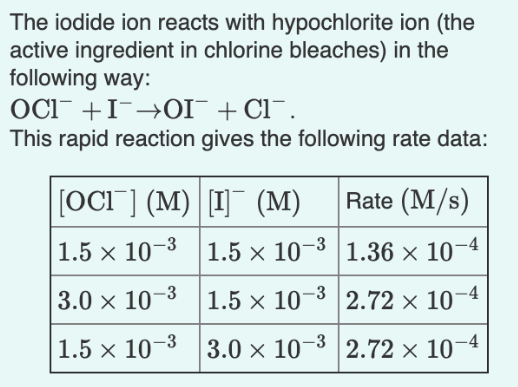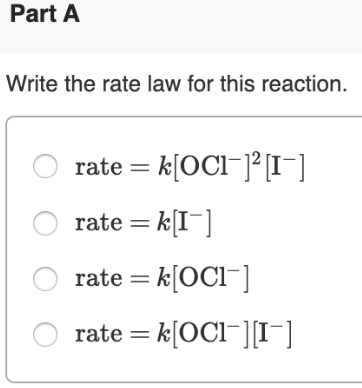# The iodide ion reacts with hypochlorite ion (the active ingredient in chlorine bleaches) in the following way: OCl^-+ I^- → OI^- + Cl^-. The rapid reaction gives the following rate data: Write the rate law for this reaction. a) rate = k[OCl^-]^2[I^-] b) rate = k[I^-] c) rate = k[OCl^-] d) rate = k[OCl^-][I^-]